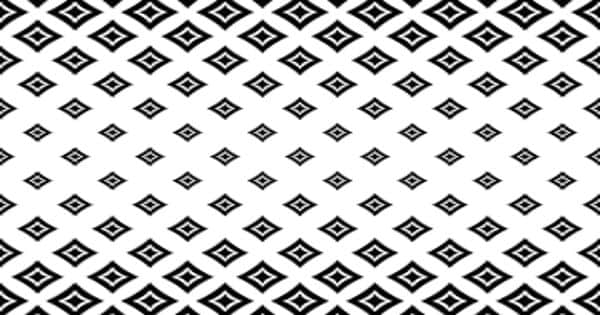# Quantum Magic Squares – the magic of mathematics

Quantum Magic Squares – the magic of mathematics

Physicists introduced the notion of the quantum magic square. The magic squares reflect the magic of mathematics. The magic of mathematics is particularly reflected in magic squares. Magic squares belong to the imagination of humanity for a long time. Magic Squares are square grids with a special arrangement of numbers in them. The oldest known magic square comes from China and is over 2000 years old. One of the most famous magic squares can be found in Albrecht Dürer’s copper engraving Melencolia I. Another one is on the facade of the Sagrada Família in Barcelona. A magic square is a square of numbers such that every column and every row sums to the same number. For example, in the magic square of the Sagrada Família every row and column sums to 33. These numbers are special because every row, column, and diagonal adds up to the same number.

In a new paper in the Journal of Mathematical Physics, Tim Netzer and Tom Drescher from the Department of Mathematics and Gemma De las Cuevas from the Department of Theoretical Physics have introduced the notion of the quantum magic square, which is a magic square but instead of numbers, one puts in matrices.

In the study, physicists show that quantum magic squares cannot be as quickly characterized as their “classical” cousins. If the magic square can contain real numbers, and every row and column sums to 1, then it is called a doubly stochastic matrix. One particular example would be a matrix that has 0’s everywhere except for one 1 in every column and every row. This is called a permutation matrix. A famous theorem says that every doubly stochastic matrix can be obtained as a convex combination of permutation matrices. In other words, this means that permutation matrices “contain all the secrets” of doubly stochastic matrices–more precisely, that the latter can be fully characterized in terms of the former. More precisely, quantum magic squares are not convex combinations of quantum permutation matrices. More precisely, quantum magic squares are not convex combinations of quantum permutation matrices.

In a new paper in the Journal of Mathematical Physics, Tim Netzer and Tom Drescher from the Department of Mathematics and Gemma De las Cuevas from the Department of Theoretical Physics have introduced the notion of the quantum magic square, which is a magic square but instead of numbers, one puts in matrices. Tim Netzer and Tom Drescher from the Department of Mathematics and Gemma De las Cuevas from the Department of Theoretical Physics have introduced the quantum magic square, which is a magic square. Still, instead of numbers, one puts in matrices. This is a non-commutative, and thus quantum, a generalization of a magic square. The authors show that quantum magic squares cannot be as easily characterized as their “classical” cousins.

“This is the general theme when generalizations to the non-commutative case are studied,” explains Tom Drescher.